# Calculator

sprinkles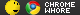2009 Sep 6 • 2547
10 ₧
Down Rodeo said:
Ah, um, yes? :p

I'm sure it makes sense. Your code looks extensible as well, for instance, the prime numbers could
be replaced by a more complete list. If you restructure a bit you can remove the goto.

If I restructeireor it a bit it would look like
code

'''<summary>
''' the radican_check is meant to abort getting the square root of the radican
''' then you know that you cannot get the square root of a negative number
''' --this is not equaviliant to -√(radical) that is possible
''' so you need to drop the negative and include an i (i as imaginary number) in front
''' of the radical thus i√(radican) however Visual Basic does not account for that
''' and does not throw an error (as they do when you try to divide by 0)
''' it simply returns 0 (not null or nothing) thus you will get 0/denominator
''' where denominator is the 2*A and 0 is x1 (ie if A=1 B=24 C=144 the end result would be -12/2 because (-1*b) is not inside the √())
''' it is not -24/2 because below that we divide the numerator by the denominator (-24/2 = -12/2)
'''</summary>

Shitty ass hotel wireless internet!!!
It's so slow it posted 31 times!!!!≡ 2009 Dec 22 at 10:06 PST — Ed. 2009 Dec 22 at 10:11 PSTsprinkles2009 Sep 6 • 2547
10 ₧
UPDATE

code
Public Function calcformula() a = INa.Text b = INb.Text c = INc.Text discriminant = (b * b) - 4 * (a * c) 'lets hope you computer knows oder of operations If discriminant < 0 Then 'the discriminant is less than 0 -see summary discriminant = Abs(discriminant) 'get the absolute value of the discriminant I = True 'remember to inlcude the I in the final answer End If discriminant_remainder = Sqrt(discriminant) If discriminant_remainder = 0 Then x1 = (-1 * b) + Sqrt(discriminant) 'discriminant should now be positive and not result in 0 x2 = (-1 * b) - Sqrt(discriminant) 'discriminant should now be positive and not result in 0 divisor_x1 = 2 * a divisor_x2 = 2 * a remainder = IEEERemainder(x1, divisor_x1) ' have to check if the complex numbers are functioning correctly If remainder = 0 Then ' have to fully reduce numbers x1 = x1 / divisor_x1 ' if cannot solve for square root and NOT get decimal leave as √() with I if applicable divisor_x1 = divisor_x1 / divisor_x1 End If remainder = IEEERemainder(x2, divisor_x2) If remainder = 0 Then x2 = x2 / divisor_x2 divisor_x2 = divisor_x2 / divisor_x2 End If End If If discriminant_remainder <> 0 Then b = (-1 * b) Return b And discriminant ElseIf errorMsg = "" Then Return x1 And x2 And divisor_x1 And divisor_x2 Else Return errorMsg End If End Function '''<summary> ''' the discriminant check (Function check()) is meant to abort getting the square root of the discriminant ''' beause the discriminant is less than 0 meaning √(-discriminant) if you remember radicals ''' then you know that you cannot get the square root of a negative number ''' --this is not equaviliant to -√(radical) that is possible ''' so you need to drop the negative and include an i (i as imaginary number) in front ''' of the radical thus i√(discriminant) however Visual Basic does not account for that ''' and throws the error NaN (Not a Number) thus you will get 0/denominator ''' where denominator is the 2*A and 0 is x1 (ie if A=1 B=24 C=144 the end result would be -12/2 because (-1*b) is not inside the √()) ''' it is not -24/2 because below that we divide the numerator by the denominator (-24/2 = -12/2) '''</summary>

code
Public Function check() If Not I = True Then ' If Not I=True must be here first because if I is true than we changed the value to get the correct square root so the rest of the function would be corrupt If discriminant < 0 Then ' If the discriminant is less than zero then there should be 1 repeated real number check if that is true If x1 <> x2 Then errorMsg = "Something went wrong" 'if x1 does not equal x2 then something went wrong report it End If If discriminant > 0 Then ' If the discriminant is greater than zero then there should be 2 different real numbers check if that is true If x1 = x2 Then errorMsg = "Something went wrong" 'if x1 does equal x2 then something went wrong report it End If End If If I = True Then End If If errorMsg = "" Then Return 0 Else Return errorMsg End If End Function

code
Private Sub Bcalculate_Click(ByVal sender As System.Object, ByVal e As System.EventArgs) Handles Bcalculate.Click If EquationToolStripMenuItem.Checked = True Then calcequation() ElseIf FormulaToolStripMenuItem.Checked = True Then calcformula() End If If I = True Then OUTx.Text = x1.ToString() + "/" + divisor_x1.ToString() + "i" + " " + x2.ToString() + "/" + divisor_x2.ToString() + "i" ElseIf errorMsg <> "" Then OUTx.Text = errorMsg ElseIf discriminant_remainder <> 0 Then OUTx.Text = b.ToString() + " +- " + "√(" + discriminant.ToString() + ")" Else OUTx.Text = x1.ToString() + "/" + divisor_x1.ToString() + " " + x2.ToString() + "/" + divisor_x2.ToString() End If End SubAll I have to do is add something to determine if it is actually
a quadratic and return a value if it is not.≡ 2009 Dec 22 at 11:35 PST — Ed. 2009 Dec 22 at 11:44 PSTDown Rodeo
Cap'n Moth of the Firehouse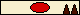2007 Oct 19 • 5486
57,583 ₧
Well, I'm very impressed, your code is a whole lot cleaner and goddamn if it doesn't check itself for errors as well. On top of that it seems to work. Well done sah!
 ≡ 2009 Dec 22 at 17:14 PSTSRAW
Rocket Man2007 Nov 6 • 2525
601 ₧
what the hell is a radican?
 ≡ 2009 Dec 22 at 19:50 PSTSRAW
Rocket Man2007 Nov 6 • 2525
601 ₧
and why would you need to check if it is a quad equation if the guy just inputs a, b and c? or just check if the guy inputs a string...................................................................................................................................................................................................................................................................................................................................................................................................................?>?>?>?>?>>>?>?>?>?>??>?>?>?>??>?>?>?
 ≡ 2009 Dec 22 at 19:52 PSTDown Rodeo
Cap'n Moth of the Firehouse2007 Oct 19 • 5486
57,583 ₧
The radican is the bit being square-rooted in a square root.
SRAW said:
and why would you need to check if it is a quad equation if the guy just inputs a, b and c?

Because of the midgets. No, if the user puts a = 0 then it's not a quadratic.
 ≡ 2009 Dec 23 at 02:21 PSTsprinkles2009 Sep 6 • 2547
10 ₧
I am also going to put a nice little bit in there to put into the equation to see if it equals 0.

And apparently there is an alternative quadratic equation with C on top?≡ 2009 Dec 23 at 06:34 PST — Ed. 2009 Dec 23 at 06:35 PSTDown Rodeo
Cap'n Moth of the Firehouse2007 Oct 19 • 5486
57,583 ₧
 ≡ 2009 Dec 23 at 06:53 PSTsprinkles2009 Sep 6 • 2547
10 ₧
Down Rodeo said:

So A can be zero? if you use the alt form?

Now its scientific notation time.

Oh no its polynomial time.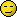I jus' realized I am putting in all these auxilairy features of
the calculator and it cannot even add or subtract!
Thats right I haven't even coded it to do basic functions.
My main problem was that I had 1 inputbox [because 2 is jus' gay]
and I didn't want to have to go through the problem of separating
functions [ie 4-3+18 would be 4 - 3 + 18 5 different strings/ints]
then I realized I could jus' get the value of the inputbox
when the pressed a function.
So lets say they input 43 then they hit minus I could get the
current value of the inputbox and then add the + then get the
current input of the inputbox again compare it with the previous
one and remove any similarities [so that 43 =? 43+ would result in
43 and +] with a few tweaks and adjustments it would work even
better!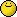≡ 2009 Dec 23 at 07:39 PST — Ed. 2009 Dec 23 at 08:00 PSTDown Rodeo
Cap'n Moth of the Firehouse2007 Oct 19 • 5486
57,583 ₧
 ≡ 2009 Dec 23 at 08:23 PSTsprinkles2009 Sep 6 • 2547
10 ₧
Oh, I also want to put in a nice little demonstration of how to
correctly use the quadratic formula. The thing is though its pretty
bland without audio, and I am not one to narrate. So DR you want to
narrate on how to do some quadratics for me?≡ 2009 Dec 23 at 12:45 PST — Ed. 2009 Dec 23 at 12:46 PSTDown Rodeo
Cap'n Moth of the Firehouse2007 Oct 19 • 5486
57,583 ₧
Not particularly, I'm very microphone shy. I've also been told I mumble.
 ≡ 2009 Dec 23 at 13:01 PSTsprinkles2009 Sep 6 • 2547
10 ₧
Then who wants to narrate?≡ 2009 Dec 23 at 14:14 PSTSolidKAYOS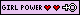2007 Jun 24 • 84
68 ₧
I got a microphone ill do it if need be.But you gatta type out what i need to say lol. If u need me too my email is triple_edgesk@yahoo.com or just tell me in general section cause i dont check here often.
Make awkward sexual advances, not war.
Down Rodeo said:
Dammit, this was the one place that didn't have this, but noooooo, molkman pisses all over that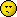≡ 2009 Dec 23 at 17:50 PST — Ed. 2009 Dec 23 at 17:54 PSTSRAW
Rocket Man2007 Nov 6 • 2525
601 ₧
dont ask havokk! she has a girly voice, just use the microsoft speech thingy
 ≡ 2009 Dec 23 at 22:36 PSTsprinkles2009 Sep 6 • 2547
10 ₧
Down Rodeo said:
Well, I'm very impressed, your code is a whole lot cleaner and goddamn if it doesn't check itself for errors as well. On top of that it seems to work. Well done sah!

I should have put in a statement or expression that checks the calculation again and fixed any errors. Also, I should have check, constantly, for overflow errors.

And, if it truly was user input error I should have had a try{} catch{} statement to handle that.

Moreover, I could have had several catches (not to be confused with the try{} catch{}) to find out where, if anything, something went wrong; and, possibly fix it, or at least, report exactly what went wrong.

Lastly, I should have included some kind of error reporting.≡ 2010 Jan 23 at 15:41 PST — Ed. 2010 Jan 23 at 19:59 PSTSolidKAYOS2007 Jun 24 • 84
68 ₧
So....How was the audio?
Make awkward sexual advances, not war.
Down Rodeo said:
Dammit, this was the one place that didn't have this, but noooooo, molkman pisses all over that≡ 2010 Jan 24 at 22:47 PSTsprinkles2009 Sep 6 • 2547
10 ₧
I never finished the project. I listened to the audio, and it wasn't bad. I may have you do more audio for me in the future, if you're up to it.≡ 2010 Jan 24 at 23:50 PST — Ed. 2010 Jan 24 at 23:50 PSTSolidKAYOS2007 Jun 24 • 84
68 ₧
sprinkles said:
I never finished the project. I listened to the audio, and it wasn't bad. I may have you do more audio for me in the future, if you're up to it.

I'm up for it. I wanna get used to talking into a mic anyways.
Make awkward sexual advances, not war.
Down Rodeo said:
Dammit, this was the one place that didn't have this, but noooooo, molkman pisses all over that≡ 2010 Jan 25 at 14:01 PSTsprinkles2009 Sep 6 • 2547
10 ₧
Good in a few days I will have a project for you.≡ 2010 Jan 25 at 18:07 PSTPage 1